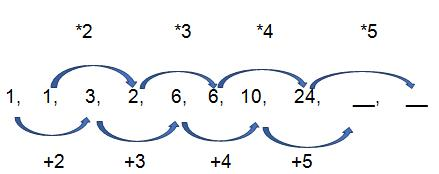Courses

# Test: Number Series Type 3 & Type 4

## 10 Questions MCQ Test Logical Reasoning (LR) and Data Interpretation (DI) | Test: Number Series Type 3 & Type 4

Description
This mock test of Test: Number Series Type 3 & Type 4 for CAT helps you for every CAT entrance exam. This contains 10 Multiple Choice Questions for CAT Test: Number Series Type 3 & Type 4 (mcq) to study with solutions a complete question bank. The solved questions answers in this Test: Number Series Type 3 & Type 4 quiz give you a good mix of easy questions and tough questions. CAT students definitely take this Test: Number Series Type 3 & Type 4 exercise for a better result in the exam. You can find other Test: Number Series Type 3 & Type 4 extra questions, long questions & short questions for CAT on EduRev as well by searching above.
QUESTION: 1

Solution:

The pattern is:
59+(1*2) = 61
61+(2*3) = 67
67+(3*4) = 79
99+(4*5) = 99
99+(5*6) = 129

QUESTION: 2

### 149, 137, 127, 109,  __

Solution:

The pattern is:
All the numbers are decreasing prime numbers in the alternate order.

QUESTION: 3

### 2.5, 1.5, 3.75, 5.625, ____

Solution:

The pattern is :
All the numbers are product of previous two numbers.
So, 5.625 * 3.75 = 21.09375

QUESTION: 4

16, 22, 26, 38, 62, ____

Solution:

Thepattern is :
16+(1*6) = 22
22+(2*2) = 26
26+(6*2) = 38
38+(3*8) = 62
62+(6*2) = 74

QUESTION: 5

1, 1, 3, 2, 6, 6, 10,  24, __, ___

Solution:QUESTION: 6

4     2     2      3     6    15    45
36   a    b      ?     d     e      f

Solution:

4 * .5 = 2
2 * 1 = 2
2 * 1.5 = 3
3 * 2 = 6
6 * 2.5 = 15
15 * 3 = 45

36 * .5 = 18
18 * 1 = 18
18 * 1.5 = 27
27 * 2 = 54
54 * 2.5 = 135
135 * 3 = 405
Therefore, number is 27

QUESTION: 7

243   81   54   54   72   120
81    a      b      c      ?      e

Solution:

The pattern is:
243*1/3 = 81
81*2/3  = 54
54*3/3  = 54
54*4/3  = 72
72*5/3  = 120

81*1/3 = 27
27*2/3  = 18
18*3/3  = 18
18*4/3  = 24
24*5/3  = 40
Therefore, number is 24

QUESTION: 8

11     15    24     40    65     101
44     a       b       c      ?        e

Solution:

11 + (2)2 = 15
15 + (3)2 = 24
24 + (4)2 = 40

40 + (5)2 = 65
65 + (6)2 = 101
Difference between 11 and 44 is of 33.
Therefore, number is 65+33 = 98

QUESTION: 9

1     3      9     31    129     651
2     a      b      c      d          ?

Solution:

The solution is :
1 * 1 + 2 = 3
3 * 2 + 3 = 9
9 * 3 + 4 = 31
31 * 4 + 5 = 129
129 * 5 + 6 = 651

2 * 1 + 2 = 4
4 * 2 + 3 = 11
11 * 3 + 4 = 37
37 *4 + 5 = 153
153 * 5 + 6 = 771
Therefore, number is 771

QUESTION: 10

15    9      8    12    36   170
19     a     ?     c     d       e

Solution:

The pattern is :
(15 – 6) * 1 = 9
(9 – 5) * 2 = 8
(8 – 4) * 3 = 12
(12 – 3) * 4 = 36
(36 – 2) * 5 = 170

(19 – 6) * 1 = 13
(13 – 5) * 2 = 16
(16 – 4) * 3 = 36
(36 – 3) * 4 = 132
(132 – 2) * 5 = 650
Therefore, number is 16